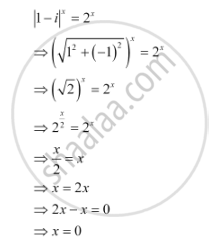# Find the number of non-zero integral solutions of the equation |1-i|x =2x. - Mathematics

Sum

Find the number of non-zero integral solutions of the equation |1-i|^x  = 2^x.

#### SolutionThus, 0 is the only integral solution of the given equation. Therefore, the number of non-zero integral solutions of the given equation is 0.

Concept: Algebra of Complex Numbers
Is there an error in this question or solution?

#### APPEARS IN

NCERT Class 11 Mathematics
Chapter 5 Complex Numbers and Quadratic Equations
Q 18 | Page 113